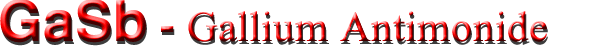Electrical properties

Basic Parameters
Mobility and Hall Effect
Transport Properties in High Electric Fields
Impact Ionization
Recombination Parameters

Basic Parameters

 Breakdown field ≈5·104 Mobility electrons ≤ 3000 cm2 V-1 s-1 Mobility holes ≤ 1000 cm2 V-1 s-1 Diffusion coefficient electrons ≤ 75 cm2/s Diffusion coefficient holes ≤ 25 cm2/s Electron thermal velocity 5.8·105 m/s Hole thermal velocity 2.1·105 m/s

Mobility and Hall Effect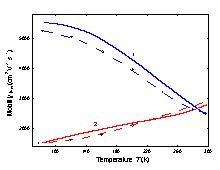Electron Hall mobility versus temperature for different doping levels. 1. Nd= 1.7·1018 cm-3 2. Nd= 2.8·1017 cm-3 Broken curves represent the experimental data. Continuous curves represent theoretical calculations. (Mathur and Jain (1979)).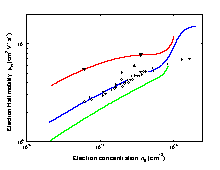Electron Hall mobility versus electron concentration no. T=77 K. Open circles represent measurements with a group of samples having approximately the same residual acceptor concentrations Na. Full symbols:specimens with lower residual acceptor concentrations. Solid lines represent the theoretical calculations for different values of compensating acceptor densities - either singly (Na-) or doubly (Na--) ionized. 1. Na- = 1.2·1017 or Na-- = 0.4·1017 cm-3 2. Na- =2.85·1017 or Na-- =0.95·1017 cm-3 3. Na- = 4.5·1017 or Na-- = 1.5·1017 cm-3 (Baxter et al. (1967)).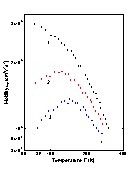Hole Hall mobility versus temperature at different compensation levels. 1. Na= 1.39·1017 cm-3; Nd= 9·1015 cm-3; 2. Na= 1.3·1017 cm-3; Nd= 9.5·1016 cm-3; 3. Na= 1.1·1017cm-3; Nd= 9.5·1016 cm-3 (Nakashima (1981)).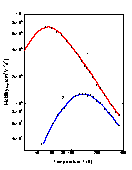Temperature dependence of hole Hall mobility. MBE technique. Hole concentration at 300 K: 1. - 2.28·1016 cm-3; 2. - 1.9·1019 cm-3. (Johnson et al. (1988)).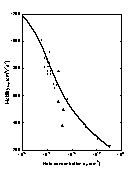The hole Hall mobility versus hole concentration, 300 K. Experimental data are taken from five different papers (Wiley (1975)).

Transport Properties in High Electric Fields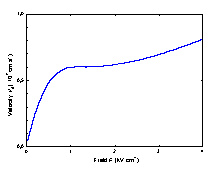Calculated field dependence of the electron drift velocity, 300 K. (Ikoma et al. (1980)).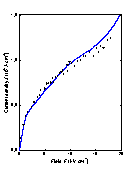Calculated (solid) end experimental (points) current density dependencies versus the electric field, 300 K. (Jantsch and Heinrich (1971)).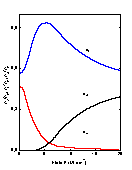Fraction of electrons in Γ, L, X valleys as a function of electric field,300 K n=6.8·1016 cm-3 (Jantsch and Heinrich (1971)).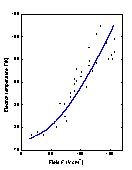Electron temperature as a function of the electric field, T=77 K. full and open circle - experimental data curve are calculated (Jantsch and Heinrich (1971)).

Impact Ionization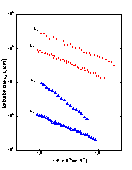The dependences of αi and βi> versus 1/F. T=77 K Open symbols : F (111). Filled symbols : F (100). (Zhingarev et al. (1981)).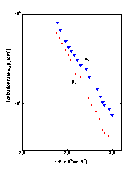The dependences of αi and βi versus 1/F). T=300 K F (100). (Hildebrand et al. (1980)).

Recombination Parameters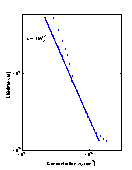Radiative lifetime versus donor concentration, T =77 K, GaSb(Te). To extract these dependences from experimental data the values of internal quantum efficiency η were taken: open circles η=0.8; filled circles η=1; (Agaev et al. (1984)).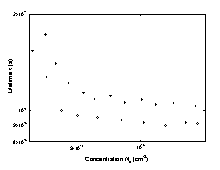Nonradiative lifetime versus donor concentrations, T =77K, GaSb(Te). open circles η= 0.8; filled circles η= 1; (Agaev et al. ).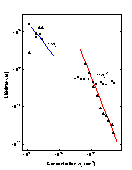Electron radiative (triangles) and nonradiative (squares) lifetime versus acceptor concentration, p-GaSb, T=77 K. (Titkov et al. (1986)).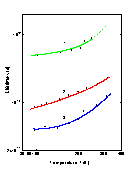Electron lifetime versus temperature at different acceptor concentrations. Na (cm-3): 1. 5·1018; 2. 2.2·1019; 3. 3.5·1019. (Titkov et al. (1986)).
 Radiative recombination coefficient ~10-10 cm3 s-1 Auger coefficient 77K 2·10-29 cm6s-1 300 K 5·10-30 cm6s-1